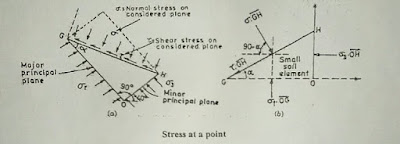Mohr Coulomb Failure Theory(Criterion) -Soil - Civil Engineering

## Mohr-Coulomb Failure Theory(Criterion)

The failure of a soil mess is more nearly in accordance with the principle of  Mohr theory of failure. The theory was first expressed by Coulomb and latter generalized by Mohr.

### Algebraically Equation

Mohr-Coulomb Failure theory can be expressed algebraically by the following equation.
𝝉 = s = f (σ) .............(1)
s =Shear stress on failure plane, at failure = Shear resistance of material & F (σ) = Function of  normal stress.

If the normal and shear stress corresponding to failure is plotted then a curve is obtained which is called the strength envelope. Coulomb defined the function F (a) as a linear function of normal stress (a) and gave the following equation.

s = c + tanφ ...........(2)
Where, c represents the intercepts on the shear axis as shown in the figure (a) & φ represents the slope of the strength line of equation (2).Figure (a) shows the Coulomb's envelope which is the graphical representation of equation (2).Mohr-Coulomb Failure Theory - Soil
Latter Mohr generalizes the above theory. He says that though there is a definite relationship between sσ, but the relation is non-linear.
He also states that the relationship between s σ depends upon the principal stresses (σ1,σ3), angle of internal friction (φ) and the inclination of failure plane.

Figure (b) shows the Mohr's envelope which is the graphical representation of equation (1). The curved failure envelope of Mohr is often referred to as straight line for most of the calculations of stability of soil mass(Considering φ is constant).